RUSSIAN JOURNAL OF EARTH SCIENCES, VOL. 20, ES4002, doi:10.2205/2020ES000730, 2020

# Classification of internal waves shoaling over slope-shelf topography

K. Terletska1, B. H. Choi2, V. Maderich1, T. Talipova3

1Institute of Mathematical Machine and System Problems, Kyiv, Ukraine

2Sungkyunkwan University, Suwon, Republic of Korea

3Institute of Applied Physics RAS, Nizhny Novgorod, Russian Federation

### Abstract

The shoaling of an internal solitary waves of depression in two layer fluid with a idealized slope-shelf topography is studied to classify the regimes of shoaling. Two mechanisms were assumed to be essential during wave shoaling: (i) wave breaking resulting in mixing and (ii) changing of the polarity of the initial wave of depression over the slope into wave of elevation on the shelf. Proposed three-dimensional $\alpha\beta\gamma$ classification diagram is based on three parameters: the slope angle $\gamma$, the non-dimensional wave amplitude $\alpha$ (wave amplitude normalized on the upper layer thickness) and the blocking parameter $\beta$ that is the ratio of the height of the bottom layer on the the shelf to the incident wave amplitude. Relations between the parameters $\alpha$,$\beta$,$\gamma$ for each regime were obtained using the empirical condition for wave breaking and weakly nonlinear theory for criterion of changing polarity of the wave. Four zones were separated in $\alpha\beta\gamma$ classification diagram: (I) Without changing polarity and wave breaking; (II) Changing polarity without breaking; (III) Wave breaking without changing polarity; (IV) Wave breaking with changing polarity. The results of field, laboratory and numerical experiments were compared with proposed classification and good agreement was found.

### 1. Introduction

The internal solitary waves (ISW) shoal and dissipate as they cross abrupt changes of the topography in the coastal ocean, estuaries and in the enclosed water bodies. Typical of the coastal ocean is the presence of a relatively shallow shelf connected to the abyssal by a continental slope. The continental slope and shelf are important sink of the internal tide energy which is dissipated due the tide generated ISWs [Lamb, 2014a]. Shoaling of ISW may results in the resuspension and transport of bottom deposits [Boegman and Stastna, 2019; Pomar et al., 2012]. The internal bores propagating into the shallows and resuspending seabed pollutants may have serious ecological consequences. ISWs with trapped core can also transport masses of water and marine organisms for some distance [Lamb, 2014a]. The transport of cold, low oxygen waters results in nutrient pumping. These facts require understanding and prediction of the ISWs transformation over coastal ocean topography to identify hot spots of wave energy dissipation and corresponding environmental implications.

Two shoaling mechanisms are important: (i) wave breaking that results in mixing and dissipation, (ii) changing of the polarity of the initial wave of depression on the slope. Wave breaking is associated with gravitational instability due to the wave overturning and shear instability. The breaking regimes over slope were classified by [Boegman et al., 2005] into plunging breakers, collapsing breakers and surging breakers assuming analogy with shoaling surface waves. The internal form of Iribarren number as the ratio of the slope of the bottom to the square root of the slope of the wave (amplitude divided by wavelength) was used for identification of breaker type. Shoaling on slope-shelf is more complicated process because additional factor appears: slowly varying depth of the shelf which affects the processes of ISW breaking and polarity change. These processes were studied in many coastal locations (e.g. [Fu et al., 2016; Moum et al., 2003; Nam and Send, 2010; Osborne et al., 1980; Orr and Mignerey, 2003; Vlasenko et al., 2014]), laboratory experiments [Wessels and Hutter, 1996], using weakly-nonlinear models ([Grimshaw et al., 2004; Helfrich and Melville, 1986; Helfrich et al., 1984; Lamb and Xiao, 2014b]) and fully nonlinear numerical models [Lamb and Xiao, 2014b; Maderich et al., 2010, 2012; Talipova et al., 2013; Vlasenko and Hutter, 2002; Vlasenko et al., 2005]. The results of these studies confirm importance of shelf in the ISW transformation. However, the available data are not generalized in the form of a classification scheme that takes into account the main features of the topography of the slope-shelf and incident internal waves.

Our goal is to develop simple classification scheme of ISW shoaling on slope-shelf based on criteria of the wave breaking and changing of polarity. The field and laboratory measurement data together with numerical modelling data are described in Section 2. Criteria of breaking and polarity change are presented in Section 3. The classification of regimes of ISW transformation over slope-shelf topography is presented in Section 4. This classification was verified in Section 5. The results are summarized in Conclusions.

### 2. Data and Methods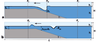Figure 1

The continuously stably stratified oceans and lakes with relatively thin pycnocline often can be approximated by the two layers of depths $h_1$ and $h_2$ with corresponding densities $\rho_1$ and $\rho_2$ (Figure 1). Total depth is $H = h_1+h_2$. The ISW exist in two waveforms depending on the position of the interface: internal solitary waves of elevation when interface is closer to the bottom ($h_1>h_2$) and wave of depression when interface is closer to the surface ($h_1 < h_2$). In this paper only waves of depression with an amplitude $a_{\rm in}$ propagating over an idealized slope-shelf are considered (Figure 1). It is assumed that the depth of the shelf is constant whereas continental slope depth varies linearly with an average inclination angle about $3\mbox{°}$. It can be less than $1\mbox{°}$ or as high as $10\mbox{°}$ whereas mean depth of shelf is in range of 100–400 m.

It was assumed that three parameters can be important for behaviour of the incident wave on slope-shelf: (i) slope inclination $\gamma$ (measured as angle); (ii) blocking parameter $\beta$ [Talipova et al., 2013] is the ratio of the height of the bottom layer on the the shelf $h_{2+}$ (Figure 1) to the incident wave amplitude $\mid a_{\mathrm{in}} \mid$

\begin{equation} \tag*{(1)} \beta =h_{2+}/|a_{\mathrm{in}}|; \end{equation}

(iii) nonlinearity parameter that is the ratio of the wave amplitude to the depth of upper layer

\begin{equation} \tag*{(2)} \alpha =|a_{\mathrm{in}}|/ h_{1}. \end{equation}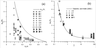Figure 2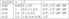Table 2

The classification is compared with available data of field observations, laboratory experiments and numerical simulations (Table 1). Parameters of simulations performed in this study are also given in Table 2 in more detail. The dependence of nonlinearity parameter $\alpha$ on normalized thickness of upper layer for waves of depression from Table 1 and Table 2 is shown in Figure 2a. The limit of ISW height in the Boussinesq approximation estimated from strongly-nonlinear theory [Choi and Camassa, 1999] is

\begin{equation} \tag*{(3)} a_{max} = \frac{h_1-h_2}{2}. \end{equation}

The corresponding curve separated area of existence of ISW. Beyond this maximum wave amplitude $|a_{\max}|$, no solitary wave solution exists.

The numerical simulations were carried out using the Navier-Stokes equations for a continuously stratified fluid. The numerical model was developed by [Kanarska and Maderich 2003] as a nonhydrostatic extension of the Princeton Ocean Model (POM). It is described in detail in [Maderich et al., 2012]. Mode-splitting technique and decomposition of pressure and velocity fields on hydrostatic and nonhydrostatic components were used in numerical method. The quasi-two-dimensional model with a resolution of 4 nodes across the wave tank was used for present calculations. No-slip boundary conditions were applied at the bottom and two end walls. The free-slip conditions were applied at the side walls. Resolution was $4500 \times 220 \times 4$ nodes for all runs.

Wave tank was of length $L = 46$ m and depth $H = 0.46$ m. The background salinity stratification at constant temperature of $20\mbox{°}$ C in the flume for both cases was modelled by two layers of thickness $h_1=0.08$ m and $h_2 = 0.38$ m separated by a thin stratified interfacial layer with thickness ($dh = 0.1$ cm) and salinity difference 28. The model was initialized using iterative solution the Dubreil-Jacotin-Long (DJL) equation [Dubreil-Jacotin, 1932] with the initial guess obtained from a weakly nonlinear theory. Stratification for all experiments remaines the same, initial amplitude, bottom slope and shelf depth were varied. Values of amplitudes of waves of $a_{\mathrm{in}}$ and parameters $\alpha, \beta, \gamma$ are given in Table 1. The DJLES spectral solver from the MATLAB package https://github.com/mdunphy/DJLES/ was used. A total of 39 runs are performed with about 12–15 runs for each incident wave amplitude (Table 2). These runs cover a range of incident ISW of depression with weakly nonlinear ($\alpha = 0.4$), moderate ($\alpha = 1$) and large amplitudes ($\alpha = 1.5$).

### 3. ISW Transformations Scenarios Over Slope-Shelf Topography

At first, consider breaking of the ISW of depression on the uniform slope. The kinematics and dynamics of breaking in the general case can depend on the slope, stratification, wave amplitude and wavelength. A several scenarios of ISW transformation can be realized. Over a mild slope a scenario of adiabatic transformation can be realized when the ISW amplitude is close to local value of $a_{\max}$ [Vlasenko et al., 2005]. Then ISW adjusts to the almost critical wave shape following depth variation. In second scenario is formation of secondary waves tail due to dispersion. One more scenario was suggested by [Maderich et al., 2012] based on numerical simulation results. In this non-adiabatic scenario, at first, shear instability arises, and then the wave changes polarity without overturning. If inclination of bottom is moderate then ISW breaks. The breaking process can be classified using the internal form of Iribarren number $Ir$ [Boegman et al., 2005] is

\begin{eqnarray*} Ir=\frac{\gamma^\ast}{\sqrt{a_{\mathrm{in}}/\lambda}}, \end{eqnarray*}

where $\gamma^\ast$ is non-dimensional slope, $\lambda$ is ISW wavelength. According this classification plunging breakers with overturning leeward face for $Ir<0.7$, collapsing breakers for $0.7 A several breaking point criteria were proposed using laboratory measurements and numerical simulations. The breaking point criterion [Vlasenko and Hutter, 2002] was build from the Navier Stokes numerical model simulations data. It was found that ratio of the amplitude of the incident wave$a_{\mathrm{in}}$to the value of undisturbed thickness of the lower layer in point where the breaking takes place,$h_b$(Figure 1a) depend on the the angle of the slope$\gamma$as \begin{equation} \tag*{(4)} \frac{a_{\mathrm{in}}}{h_b} = \frac{0.8^{\mathrm o}}{\gamma}+0.4. \end{equation} The comparison of (4) with data from Table 1 and Table 2 show good agreement except extreme case of step-like topography ($\gamma=90\mbox{°}$). Figure 2b shows relatively weak dependence of wave breaking on$\gamma$in the range$ \gamma <5\mbox{°}$, whereas wave breaking strongly depends on the slope in the case$\gamma>5\mbox{°}$. That is the consequences of dispersive effects that work simultaneously with nonlinearity. When$\gamma>5\mbox{°}$wave transforms over the slope on a short distance and then nonlinear effects dominate, while in the case$\gamma<5\mbox{°}$dispersive effects dominate and the significant part of the energy transfers into the dispersive wave trains. Another criterion was built fitting results of laboratory experiments on breaking over sloping bottom [Boegman et al., 2005] \begin{equation} \tag*{(5)} \frac{a_{\mathrm{in}}}{h_b} = \frac{0.14}{(\lambda_{\mathrm{in}}/L_i)^{0.52}}-0.3. \end{equation} where$L_i=h_{2b}/\gamma^\ast$,$\lambda_{\mathrm{in}}$is the wavelength of incident ISW. Notice, that$\lambda_{in}$was estimated from weakly-nonlinear KdV theory and$\gamma^\ast$is height to slope length ratio. This criterion was updated by [Aghsaee et al., 2010] using numerical simulation output: \begin{equation} \tag*{(6)} \frac{a_{\mathrm{in}}}{h_b} = \frac{0.14}{(\lambda_{in}/L_i)^{0.28}}+0.13. \end{equation} The difference between relations (5) and (6) can be explained by extension of range of the data and by direct estimation of wave length in [Aghsaee et al., 2010]. The maximum of interface descent$H_i$in location of the wave breaking was estimated by [Sutherland et al., 2013] as \begin{eqnarray*} H_i = \sqrt{4\gamma^\ast a_{\mathrm{in}}\lambda_{\mathrm{in}}}. \end{eqnarray*} The ISW propagation and breaking in the system slope-shelf is more complicated process because wave behaviour depends also on thickness of the lower layer$h_{2+}$over shelf. According to weakly-nonlinear theory (e.g. [Grimshaw et al., 2004]) coefficient of quadratic nonlinearity in the Gardner equation changes its sign in the point where the thicknesses of two layers become equal, while the coefficient of cubic nonlinearity is always negative. Notice that numerical experiments using full Navier-Stokes equations [Lamb and Xiao, 2014b; Maderich et al., 2010] confirm applicability of the Gardner equation to predict turning point$h_1=h_2$even for wave of large amplitude. This relation for turning point can be can be expressed through parameters$\alpha$and$\beta$using (1) and (2) \begin{equation} \tag*{(7)} \beta=1/\alpha. \end{equation} The observations [Fu et al., 2016] showed that the transition of large-amplitude IWs during the shoaling process is related to$\beta$that is good indicator of wave deformation. When$\beta<1.2$, all waves are bottom-trapped elevation waves. It was shown in the laboratory study [Cheng et al., 2011] interval$1.08<\beta< 1.78 $demarcated the boundary between non-inversion and inversion ISWs regimes. Moreover,$\beta < 1.08$might be taken to distinguish waveform inversion induced by intense wave breaking. An instability of ISW on slope-shelf [Helfrich et al., 1986] and [Cheng et al., 2011] could be classified into three scenarios: (1) no instability, (2) shear instability, and (3) overturning (breaking) and second mode wave generation. The shear instability resulting in Kelvin-Helmgoltz (KH) billows are taking place for$3.3<\beta<5$and overturning was observed for$\beta<3.3$[Cheng, 2011]. The internal waves of depression over the slope-shelf topography with slope angle about$20.5\mbox{°}$were studied in laboratory experiments by [Lim, 2008]. For the values$2.7 <\beta<7 $wave saves their form of depression and for$\beta = 1.39$wave breaks and transforms into internal surge or solitary wave train. In the limiting case of bottom step ($\gamma =90\mbox{°}$) three different regime were identified in [Talipova, 2013] for$h_2 > 0$: (i) Weak interaction$3.1<\beta$, when the wave dynamics can be fully described by weakly nonlinear theory [Grimshaw 2008]; (ii) Moderate interaction$2 <\beta< 3.1$when the mechanism for wave breaking over the step is mainly shear instability. (iii) Strong interaction when supercritical flow in the step vicinity results in a backward jet and vortices for depression waves, and in a forward moving vortex (bolus) transporting dense fluid onto the step at$\beta < 2$. ### 4. Classifications of Regimes of ISW Transformation Over Slope-Shelf Topography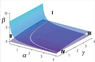Figure 3 A new classification of regimes of internal solitary wave interaction with a shelf-slope topography in the framework of two-layer fluid with layer depths$h_1$and$h_2(x)$is proposed. A three-dimensional diagram with the dependence on parameters$\alpha, \beta, \gamma$($\alpha\beta\gamma$diagram) is introduced in Figure 3 where$\alpha$is the wave amplitude normalized on the upper layer thickness. Maximal amplitude$a_{\max}$defined by (3) can be rewritten in the variables$|a_{\mathrm{in}}|/h_1$and$h_1/H$as$\alpha<0.5/(h_1/H)-1$[Aghsaee, 2010]. This ratio determines the geometric parameters of the existence of ISWs. As can be seen from Figure 2a, the nonlinear parameter$\alpha$for the majority of waves observed on the shelf varies within interval of 0.1–2. The blocking parameter$\beta$introduced in [Talipova et al., 2013] controls the energy loss due to ISW transformation over the slope-shelf topography. The slope angle$\gamma$determines breaking process. The type of ISW shoaling of depends on how wave will pass through the cross sections$x_b$and$x_r$(Figure 1). A several scenarios can be realized: • Wave breaks over the slope-shelf if$h_b > h_{2+}$; • Wave does not break over the slope-shelf$h_b < h_{2+}$; • Wave changes polarity as it transforms over the slope-shelf if$h_1>h_{2+}$; • Wave does not change polarity as it transforms over the slope-shelf if$h_1 < h_{2+}$; In$\alpha\beta\gamma$diagram the 3D space is separated by the surfaces$f_1(\beta; \gamma) = 0$and$f_2(\alpha; \beta) = 0$. The surface$f_1(\beta; \gamma) = 0$separates the region of parameters where breaking takes place from the region without breaking. The polarity change surface$f_2(\alpha; \beta) = 0$is obtained from the condition (7). In the two-layer stratification waves of depression converted into waves of elevation at the turning point ($h_2 = h_1$) as they propagate from deep water onto a shallow shelf. Thus intersecting surfaces$f_1$and$f_2$divide three-dimensional ($\alpha, \beta, \gamma$) space into four zones (Figure 3). Zone I located above these two surfaces corresponds to the non-breaking regime. Zone II is placed above breaking surface but below the surface of changing polarity. It corresponds to the regime of changing polarity without breaking. Zone III is placed above surface of changing polarity but below breaking surface. It corresponds to the regime of wave breaking without changing polarity. Zone IV located below of these two surfaces corresponds to the regime of wave breaking with changing polarity. For each slope angle$\gamma$the blocking parameter value$\beta_{\mathrm{br}}$that divide zone of non breaking regime$\beta>\beta_{\mathrm{br}}$and breaking regime$\beta<\beta_{\mathrm{br}}$. can be found from (7) using (4) at$h_{2+} = h_b$that yields \begin{equation} \tag*{(8)} \beta_{\mathrm{br}} =\gamma/(0.8+0.4\gamma) \end{equation} As seen from (8), the breaking value of the blocking parameter does not depend on the ISW nonlinearity parameter$\alpha$. We can also obtain value$\alpha_{\mathrm{br}}$that divide zone IV on breaking regime when wave first breaks and that changing polarity$\alpha>\alpha_{\mathrm{br}}$and when wave first change polarity and than breaks and breaking regime$\alpha<\alpha_{\mathrm{br}}$. It can be found from (7) using (8) that yields \begin{eqnarray*} \alpha_{\mathrm{br}} =(0.8+0.4\gamma)/\gamma. \end{eqnarray*} ### 5. Comparison of Classification With Data From Field Measurements, Laboratory Experiments and Numerical Simulations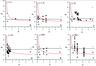Figure 4 The data from field and laboratory measurements and numerical simulations were compared in Figure 4 with proposed$\alpha\beta\gamma$diagram. These data are given for six cross-sections$\alpha\beta$at different angles of slope$\gamma$. In the figure red line corresponds to the polarity change criterion (7), whereas black line corresponds to the breaking criterion (4). The measurement and simulation data were identified as belonged to non breaking without changing polarity cases (diamonds), changing polarity without breaking cases (circles), wave breaking without changing polarity cases (crosses), and cases of changing polarity with breaking (triangles). The wave parameters are given in Table 1 and Table 2. As seen in Figure 4a, at small slope ($\gamma=1\mbox{°}$) strongly nonlinear waves of amplitude 60 m propagating in the South China Sea at ocean depth from 264 m to a depth 110 m [Orr and Mignerey, 2003] were between non-breaking waves zone I and the breaking with changing polarity (zone III). However, these waves were subjected to the shear instability. The ISW shown in Figure 4b as black symbols were data from simulations for slope ($\gamma=1.5\mbox{°}$). They transformed into the elevation waves without breaking (zone II), broken with changing polarity (zone IV) and transformed without changing polarity and wave breaking (zone I) in dependence on$\alpha$and$\beta$. The ISW transformation in the Andaman Sea [Osborne et al., 1980] marked by open symbol corresponds to the zone II where wave change polarity without breaking. Over the shelf of a slope$3\mbox{°}$(Figure 4c) large amplitude depression waves in the South China Sea [Fu et al., 2016] transformed according scenarios for zones I–IV. Notice, that observed ISW in the Celtic Sea [Vlasenko et al., 2014] is placed in vicinity of node point connecting zones I–IV. Data from laboratory experiments [Cheng, 2011] on the ISW transformation over trapezoidal topography with$\gamma=14\mbox{°}$were classified in Figure 1d. Laboratory experiments suggests that$\beta \approx1.8$might be taken for demarcating boundary between non-inversion and inversion cases. Scenario when$\beta < 1.1$was accompanied by run-down, internal hydraulic jump, vortex motion, turbulent mixing and surging up along the slope, but with different degrees of strength, from weak to moderate and strong, depending on the value of$\alpha$. For the step-like topography with slopes$60\mbox{°}$and$90\mbox{°}$we present results of simulations given in Table 2 and in [Talipova et al., 2013]. Here diamonds correspond to non-breaking and non-wave inversion regime defined by [Talipova et al., 2013] as weak interaction, crosses correspond to breaking and inversion, this case also includes cases with shear instability and finally triangles corresponds to breaking with formation of boluses over shelf.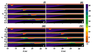Figure 5 Consider in more detail characteristic for many coastal areas case of$\gamma=1.5\mbox{°}$. In Figure 5 the evolution of cross-section of salinity$S$is shown for$\alpha=1.5$using results of numerical simulations of laboratory scale given in Table 2. Four values were used:$\beta=0.58$($h_{2+}=7$cm) (zone IV) ,$\beta$=0.8 ($h_{2+}=9.6$cm) (zone III),$\beta =1.41$($h_{2+}=17$cm) (zone I). These experiments are marked by red symbols in Figure 4b. In Figure 5 the evolution of the cross-section salinity field for scenarios of ISW transformation corresponding zones I–IV at$\gamma=1.5\mbox{°} $is shown. Three cases for same value$\alpha = 1.5$($a = 0.12$m), but with different values of$\beta$are considered:$\beta =0.58$($h_{2+} = 7$cm) (zone IV) ,$\beta = 0.8$($h_{2+} = 9.6$cm) (zone III),$\beta = 1.41$($h_{2+} = 17$cm) (zone I). These cases are marked by red symbols in Figure 4b. According the breaking criterion (4) the breaking depth$h_{b}$in this case should be$h_{b} = 12.85$cm. Therefore, when the depth of the lower layer over the shelf is greater than$h_{2+} > 12.85$cm then no breaking occurs. It is follow from (8) that blocking parameter value for breaking waves depth is$\beta > \beta_{\mathrm{br}} = 1.07$. Therefore, if$h_{2+} < 12.85$cm then wave will break on slope. Figure 5a shows that at$(\alpha, \beta, \gamma)=(1.5;1.41;1.5\mbox{°})$ISW evolves with formation of wave train without breaking and changing polarity as predicted$\alpha \:\beta \:\gamma$classification diagram (red diamond) for zone I. This evolution can be described in frame weakly-nonlinear theory (e.g. Gardner equation) in agreement. The value$\beta = 1.41$is close to value of$\beta_{\mathrm{br}} = 1.07$. However, no breaking occurs during ISW passing over the shelf. In Figure 5b the ISW breaking without changing polarity (Zone II) corresponding to$(\alpha,\:\beta,\:\gamma)=(0.25;\:1.5;\:1.5\mbox{°})$is shown. In classification diagram it marked by red circle in Figure 4b. As can be seen from 3D diagram this regime can be realized only for weakly nonlinear and moderate internal waves with$\alpha<\alpha_{cr}$. The ISW transformation with wave breaking without changing polarity (Zone III) shown in Figure 5c occurs in simulation with parameters$(\alpha,\:\beta,\:\gamma)=(1.5;\:0.8;\:1.5\mbox{°})$. It was marked as red cross in Figure 4b. This transformation is essentially nonlinear and might not occurs for small amplitude waves. The value of$\beta=0.8$was less than$\beta_{\mathrm{br}} =1.07$and breaking occurs near the shelf break, forming region of mixed fluid that is absent in the case shown in Figure 5a. Notice that value of$\beta_{\mathrm{br}} =1.07$obtained from (4) gives a good estimate for wave breaking location in these numerical simulations. Figure 5d shows case of ISW breaking with changing polarity occurs corresponding zone IV where$(\alpha,\:\beta,\:\gamma)=(1.5;\:0.58;\:1.5\mbox{°})$. It is marked as red triangle in Figure 4b. For weakly nonlinear and moderate amplitude waves with$\alpha<\alpha_{\mathrm{br}}$the waves the first changes their polarity as they moves over the slope and then break. But for large amplitude waves$\alpha> \alpha_{\mathrm{br}}$waves break firstly forming boluses, and then waves move upslope as shown in Figure 5d. ### 6. Conclusions The shoaling of an internal solitary waves in two layer fluid with a idealized slope-shelf topography was studied to classify the regimes of wave transformation. Two mechanisms were assumed to be essential during wave shoaling: (i) wave breaking resulting in mixing and (ii) changing of the polarity of the initial wave of depression over the slope into wave of elevation on the shelf. Proposed three-dimensional$\alpha\:\beta\:\gamma$classification diagram is based on three parameters: the slope angle$\gamma$, the non-dimensional wave amplitude$\alpha$(wave amplitude normalized on the thermocline thickness) and the blocking parameter$\beta$that is the ratio of the height of the bottom layer on the the shelf to the incident wave amplitude. Relations between the parameters$\alpha, \:\beta, \:\gamma$for each regime were obtained with using the empirical condition for wave breaking and weakly nonlinear theory for criterion of changing polarity of the wave. Four zones were separated in$\alpha\beta\gamma\$ classification diagram: (I) – Without changing polarity and wave breaking, (II) – Changing polarity without breaking; (III) – Wave breaking without changing polarity; (IV) – Wave breaking with changing polarity. We concluded that results of field, laboratory and numerical experiments are in good agreement with proposed classification which can be used for identification of hot spots of energy dissipation in the ocean.

#### Acknowledgment

This work is partially supported RFBR grant No 19-05-00161 (T. Talipova).

### References

Aghsaee, P., L. Boegman, K. G. Lamb (2012) , Breaking of shoaling internal solitary waves, J. Fluid Mech., 659, p. 289–317, https://doi.org/10.1017/S002211201000248X.

Boegman, L., M. Stastna (2019) , Sediment resuspension and transport by internal solitary waves, Annu. Rev. Fluid. Mech., 51, p. 129–154, https://doi.org/10.1146/annurev-fluid-122316-045049.

Boegman, L, G. Ivey N., J. Imberger (2005) , The degeneration of internal waves in lakes with sloping topography, Limnol. Oceanogr., 50, p. 1620–1637, https://doi.org/10.4319/lo.2005.50.5.1620.

Cheng, M. H., J. R.-C. Hsu, C. Y. Chen (2011) , Laboratory experiments on waveform inversion of an internal solitary wave over a slope-shelf, Environ Fluid Mech., 11, p. 353–384, https://doi.org/10.1007/s10652-010-9204-x.

Choi, W. E., R. Camassa (1967) , Fully nonlinear internal waves in a two-fluid system, J. Fluid Mech., 396, p. 1–36, https://doi.org/10.1017/S0022112099005820.

Fu, K. H., Yu. H. Wang, C. P. Lee, I. H. Lee (2016) , The deformation of shoaling internal waves observed at the Dongsha Atoll in the northern South China Sea, Coastal Engineering Jornal, 58, p. 1650001, https://doi.org/10.1142/S0578563416500017.

Grimshaw, R., E. N. Pelinovsky, T. G. Talipova, A. Kurkin (2004) , Simulations of the transformation of internal solitary waves on oceanic shelves, J. Phys. Oceanogr., 34, p. 2774–2791, https://doi.org/10.1175/JPO2652.1.

Helfrich, K., W. K. Melville, W. Miles (1984) , On interfacial solitary waves over slope-shelf topography, J. Fluid Mech., 149, p. 305–317.

Helfrich, K. R., W. K. Melville (1986) , On long nonlinear internal waves over slopeshelf topography, J. Fluid Mech., 167, p. 285–308, https://doi.org/10.1017/S0022112086002823.

Kanarska, Yu., V. Maderich (2003) , A non-hydrostatic numerical model for calculating of free-surface stratified flows, Ocean Dynamics, 51, p. 176–185.

Kao, T. W., F. Pan S., D. Renouard (2010) , Internal solitions on the pycnocline: generation, propagation, shoaling and breaking over a slope, J. Fluid Mech., 159, p. 19–53, https://doi.org/10.1063/1.3455984.

Lamb, K. G. (2014a) , Internal wave breaking and dissipation mechanisms on the continental slope/shelf, Annu. Rev. Fluid Mech., 46, p. 231–254, https://doi.org/10.1146/annurev-fluid-011212-140701.

Lamb, K. G., W. Xiao (2014b) , Internal solitary waves shoaling onto a shelf: comparisons of weakly-nonlinear and fully nonlinear models for hyperbolic-tangent stratifications, Ocean Model., 78, p. 17–34, https://doi.org/10.1016/j.ocemod.2014.02.005.

Lim, K., G. N. Ivey, R. I. Nokes (2008) , The generation of internal waves by tidal flow over continental shelf/slope topography, Environ Fluid Mech., 8, p. 511–526, https://doi.org/10.1007/s10652-008-9085-4.

Maderich, V., T. Talipova, et al. (2010) , Interaction of a large amplitude interfacial solitary wave of depression with a bottom step, Physics of Fluids, 22, p. 1–36.

Maderich, V., I. Brovchenko, et al. (2012) , Numerical simulations of the nonhydrostatic transformation of basin-scale internal gravity waves and wave-enhanced meromixis in lakes, Nonlinear internal waves in lakes, K. Hutter (Ed.), p. 192–276, Springer, Berlin, Heidelberg, https://doi.org/10.1007/978-3-642-23438-5_4.

Moum, J. N., D. M. Farmer, W. D. Smyth, et al. (2003) , Structure and generation of turbulence at interfaces strained by internal solitary waves propagating shoreward over the continental shelf, J. Phys. Oceanogr., 33, p. 2093–2112, https://doi.org/10.1175/1520-0485(2003)033%3C2093:SAGOTA%3E2.0.CO;2.

Nam, S. H., U. Send (2010) , Direct evidence of deep water intrusions onto the continental shelf via surging internal tides, J. Geophys. Res., 116, no. C05004, p. 1–15, https://doi.org/10.1029/2010JC006692.

Orr, M., P. C. Mignerey (2003) , Nonlinear internal waves in the South China Sea: observation of the conversion of depression internal waves to elevation internal waves, J. Geophys. Res., 108, p. 9–16, https://doi.org/10.1029/2001JC001163.

Osborne, A. R., T. L. Burch, B. Butman, J. Pineda (1890) , Internal solitons in the Andaman Sea, Science, 208, p. 451–460, https://doi.org/10.1126/science.208.4443.451.

Pomar, L., M. Morsilli, P. Hallock, B. Badenas (2012) , Internal waves, an under-explored source of turbulence events in the sedimentary record, Earth-Science Reviews, 111, p. 56–81.

Sutherland, B. R., K. J. Barrett, G. N. Ivey (2013) , Shoaling internal solitary waves, J. Geophys. Res., 118, p. 4111–4124, https://doi.org/10.1002/jgrc.20291.

Talipova, T., K. Terletska, V. Maderich, et al. (2013) , Internal solitary wave transformation over a bottom step: Loss of energy, Physics of Fluids, 25, p. 1620–1637.

Vlasenko, V., K. Hutter (2002) , Numerical experiments on the breaking of solitary internal waves over a slope shelf topography, J. Phys. Oceanogr., 32, p. 1779–1793, https://doi.org/10.1175/1520-0485(2002)032%3C1779:NEOTBO%3E2.0.CO;2.

Vlasenko, V., L. Ostrovsky, K. Hutter (2005) , Adiabatic behavior of strongly nonlinear internal solitary waves in slope-shelf areas, J. Geophys. Res., 110, p. 289–317, https://doi.org/10.1029/2004JC002705.

Vlasenko, V., N. Stashchuk, M. E. Inall, et al. (2014) , Tidal energy conversion in a global hot spot: On the 3-D dynamics of baroclinic tides at the Celtic Sea shelf break, J. Geophys. Res., 119, p. 3249–3265, https://doi.org/10.1002/2013JC009708.

Wessels, F, K. Hutter (1996) , Interaction of internal waves with topographic sill in a two-layer fluid, J. Phys. Oceanogr., 26, p. 5–20, https://doi.org/10.1175/1520-0485(1996)026%3C0005:IOIWWA%3E2.0.CO;2.

Received 20 April 2020; accepted 25 June 2020; published 12 July 2020.Citation: Terletska K., B. H. Choi, V. Maderich, T. Talipova (2020), Classification of internal waves shoaling over slope-shelf topography, Russ. J. Earth Sci., 20, ES4002, doi:10.2205/2020ES000730.

Generated from LaTeX source by ELXfinal, v.2.0 software package.